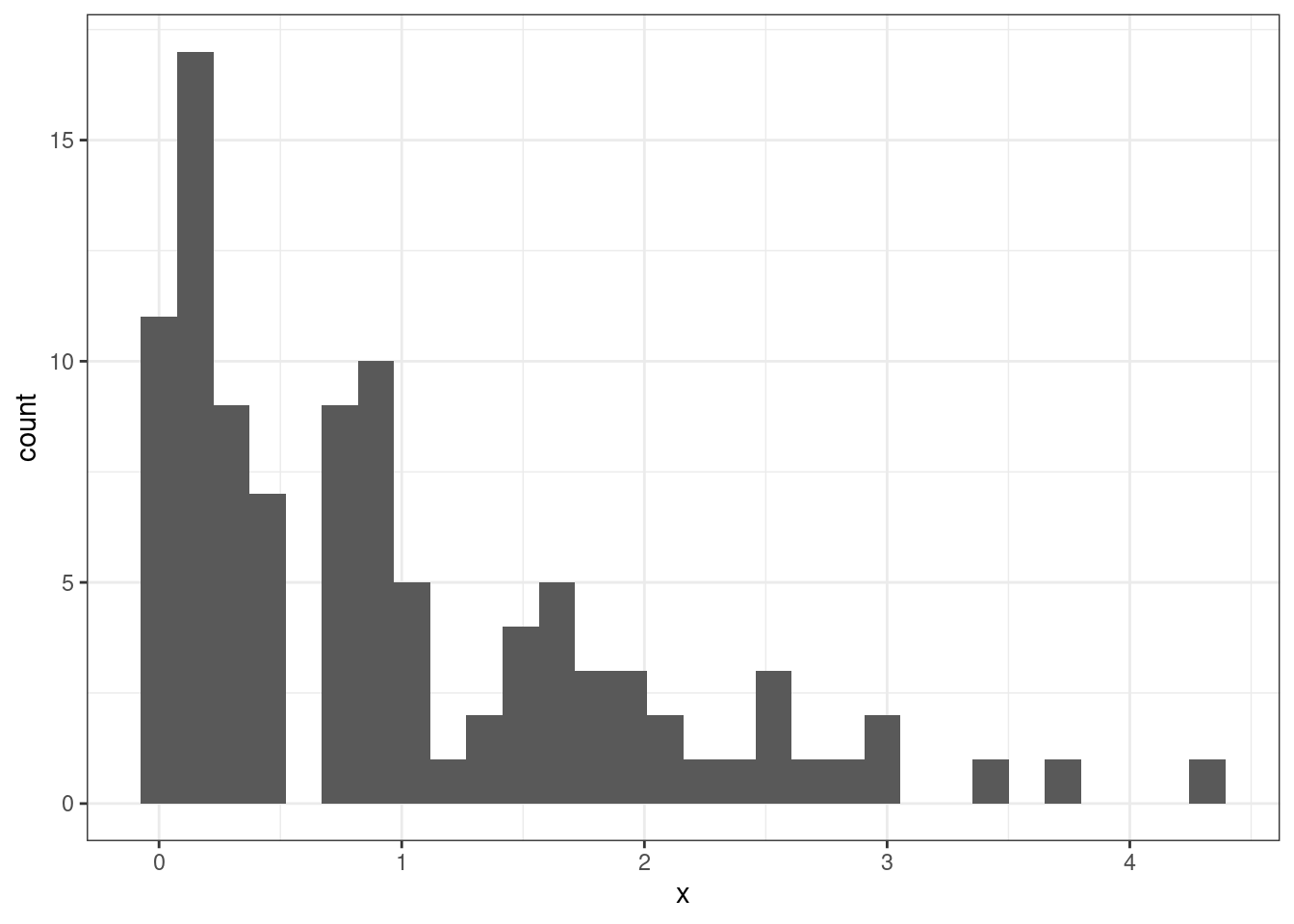# Ch. 3, Coding Question 1

library(ggplot2)
set.seed(1234) # setting the seed means that we will get the same results
x <- rexp(100) # make 100 draws from an exponential distribution

ggplot(data=data.frame(x=x),
mapping=aes(x=x)) +
geom_histogram() +
theme_bw()# Ch. 3, Extra Question 1

Part a

\begin{aligned} \mathbb{E}[Y] &= \mathbb{E}[5+9X] \\ &= \mathbb{E} + \mathbb{E}[9X] \\ &= 5 + 9\mathbb{E}[X] \\ &= 95 \end{aligned}

where the first equality holds by the definition of $$Y$$, the second equality holds because expectations can pass through sums, the third equality holds because the expectation of a constant is just the constant itself and because constants can come outside of expectations, and the last equality holds because $$\mathbb{E}[X]=10$$.

Part b

\begin{aligned} \mathrm{var}(Y) &= \mathrm{var}(5+9X) \\ &= \mathrm{var}(9X) \\ &= 81 \mathrm{var}(X) \\ &= 162 \end{aligned}

where the first equality holds by the definition of $$Y$$, the second equality holds because $$5$$ is a constant and doesn’t contribute to the variance, the third equality holds because constants can come out of the variance (after squaring it), and the last equality holds because $$\mathrm{var}(X)=2$$.

# Ch. 3, Extra Question 2

\begin{aligned} \mathrm{var}(bX) &= \mathbb{E}\big[ (bX - \mathbb{E}[bX])^2 \big] \\ &= \mathbb{E}\big[ (bX - b\mathbb{E}[X])^2 \big] \\ &= \mathbb{E}\Big[ \big(b(X-\mathbb{E}[X])\big)^2\Big] \\ &= \mathbb{E}\big[b^2 (X-\mathbb{E}[X])^2\big] \\ &= b^2 \mathbb{E}\big[(X-\mathbb{E}[X])^2\big] \\ &= b^2 \mathrm{var}(X) \end{aligned}

where the first equality holds by the definition of variance, the second equality holds because $$b$$ can come out of the inside expectation because it is a constant, the third equality factors out the $$b$$ from both terms, the fourth equality squares the inside terms, the fifth equality holds because $$b^2$$ is a constant and can therefore come out of the expectation, the last equality holds because $$\var(X) = \mathbb{E}[(X-\mathbb{E}[X])^2]$$ (which is just the definition of the variance of $$X$$).

# Ch. 3, Extra Question 3

Part a

\begin{aligned} \mathbb{E}[Y] &= \mathbb{E}[Y|X=1] \mathrm{P}(X=1) + \mathbb{E}[Y|X=0]\mathrm{P}(X=0) \\ &= \mathbb{E}[Y|X=1] \mathrm{P}(X=1) + \mathbb{E}[Y|X=0](1-\mathrm{P}(X=1)) \\ &= 5\' 4\" (0.5) + 5\' 9\" (0.5) \\ &= 5\' 6.5\" \end{aligned}

Part b

The answer from part a is related to the law of iterated expectations because the key step in that problem is to relate the overall expectation, $$\mathbb{E}[Y]$$, to the conditional expectations, $$\mathbb{E}[Y|X=0]$$ and $$\mathbb{E}[Y|X=1]$$. The law of iterated expectations says that unconditional expectations are equal to averages of conditional expectations, which is what we use in the first step of the answer for part a.

# Ch. 3, Extra Question 4

Part a

$$f_X(21) = 0.1$$. We know this because the sum of the pdfs across all possible values of $$X$$ must add up to 1.

Part b

\begin{aligned} \mathbb{E}[X] &= \sum_{x \in \mathcal{X}} x f_X(x) \\ &= 2 f_X(2) + 7 f_X(7) + 13 f_X(13) + 21 f_X(21) \\ &= 2 (0.5) + 7 (0.25) + 13 (0.15) + 21 (0.1) \\ &= 6.8 \end{aligned}

Part c

To calculate the variance, I’ll use the expression $$\mathrm{var}(X) = \mathbb{E}[X^2] - \mathbb{E}[X]^2$$. Thus, the main new thing to calculate is $$\mathbb{E}[X^2]$$:

\begin{aligned} \mathbb{E}[X^2] &= \sum_{x \in \mathcal{X}} x^2 f_X(x) \\ &= 2^2 f_X(2) + 7^2 f_X(7) + 13^2 f_X(13) + 21^2 f_X(21) \\ &= 4 (0.5) + 49 (0.25) + 169 (0.15) + 441 (0.1) \\ &= 83.7 \end{aligned}

Since, we already calculated $$\mathbb{E}[X] = 6.8$$ in part a, this implies that $$\mathbb{E}[X]^2 = 46.24$$. Thus, \begin{aligned} \mathrm{var}(X) &= 83.7 - 46.24 \\ &= 37.46 \end{aligned}

Part d

$F_X(1) = 0$

since the smallest possible value of $$X$$ is 2.

\begin{aligned} F_X(7) &= f_X(2) + f_X(7) \\ &= 0.75 \end{aligned}

\begin{aligned} F_X(8) &= f_X(2) + f_X(7) \\ &= 0.75 \end{aligned} \begin{aligned} F_X(25) &= 1 \qquad \end{aligned}

since all possible values that $$X$$ can take are less than 25.